/

### Function Description

Returns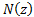, the cumulative unit Normal distribution function, i.e. for a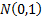distribution, where: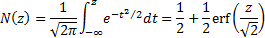The equivalent function in Microsoft Excel is NORMSDIST.

In practice, the website uses the following rational approximation to the above integral (as apparently do some versions of Microsoft Excel), source e.g. Abramowitz and Stegun (1970):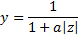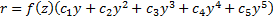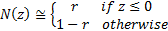where: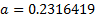,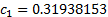,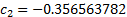,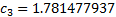,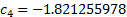,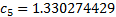and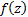is the unit Normal density function, i.e.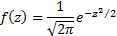Is a special case of MnProbDistCdf.

Contents | Prev | Next